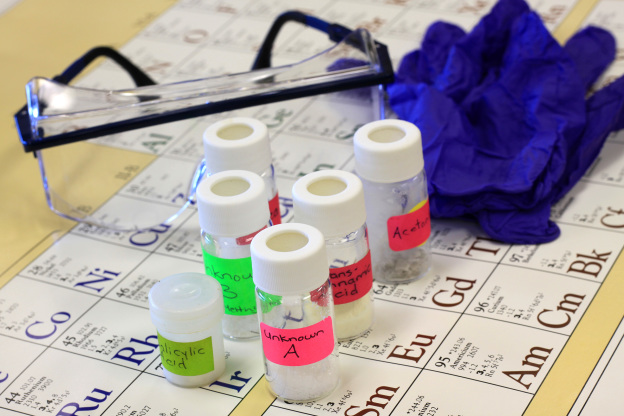# Nursing AbbreviationsWhenever you take nursing exams such as the NCLEX or HESI, you will be required to know certain nursing abbreviations. These nursing abbreviations will also be critical for you to know from your first day of nursing to your last. Below is a list of nursing abbreviations that all future and current nurses need to know.

## Nursing Abbreviations: Units of Measure

The following is a list of nursing abbreviations for units of measure:

• c = cup
• cc = cubic centimeters
• cm = centimeters
• dr = drams
• dss = 2 teaspoons
• fl = fluid
• ft = foot
• g = grams
• gal = gallon
• gl = glass
• gr = grains
• gt = drop
• gtt = drops
• in = inches
• kg = kilograms
• L = liters
• lb = pound
• m = meters
• mcg = micrograms
• mEq = milliquivalents
• mg = milligrams
• mL = milliliters
• mm = millimeters
• oz = ounce
• pt = pint
• qt = quart
• tbsp = tablespoons
• tsp = teaspoons
• U = unit

## Nursing Abbreviations: Other Abbreviations

The following is a listing of miscellaneous nursing abbreviations:

• a = before (ante)
• ABG = arterial blood gas
• ABT = antibiotic therapy
• ac = before meals (ante cibum)
• AD = right ear (auricula dexter)
• ad lib = as desired
• ADA = American Diabetes Ass.
• am = before noon (ante meridian)
• AMA = against medical advice
• aq = water
• AS = left ear (auricula sinister)
• AU = both ears (auriculi utro)
• bid = twice a day (0900, 1700)
• BP = blood pressure
• BUN = blood urine nitrogen
• c = with
• cap = capsule
• CAD = coronary artery disease
• CAT = computerized axial tomography
• CBC = complete blod count
• CF = cystic fibrosis
• CHF = congestive heart failure
• CNS = central nervous system
• CO = cardiac output
• COPD = chronic obstructive pulmonary disease
• CPK = creatinine phosphokinase
• CSF = cerebrospinal fluid
• CVA = cerebrovascular accident
• CVP = central venous pressure
• EC = enteric coated
• ECG = electrocardiogram
• EEG = electroencephalogram
• elix = elixir
• ext = extract
• GFR glomerulofiltration rate
• GT = gastrostomy
• h = hour
• hct = hematocrit
• hgb = hemoglobin
• hs = hour of sleep, bedtime (2100)
• ICP = intracranial pressure
• IM = intramuscular
• IV = intravenous
• IVP = intravenous push/pyelogram
• IVPB = intravenous piggyback
• KVO = keep vein open
• MI = myocardial infarction
• NG = nasogastric
• NJ = nasojejunal
• NPO = nothing by mouth
• NS = normal saline
• OD = right eye (oculus dexter)
• oint = ointment
• OTC = over the counter
• OS = left eye (oculus sinister)
• OU = both eyes (oculo utro)
• p = after (post)
• pc = after meals (post cibum)
• per = by
• pm = after noon (post meridian)
• po = by mouth (per os)
• pr = per rectal
• prn = whenever necessary
• PT = prothrombin time
• PTT = partial prothrombin time
• q = every
• q1h = every 1 hour
• q2h = every 2 hours
• q3h = every 3 hours
• q4h = every 4 hours (0900, 1300, 1700,…0500)
• q6h = every 6 hours (2400, 0600, 1200, 1800)
• q8h = every 8 hours (0600, 1400, 2200)
• qd = every day (0900)
• qh = every hour
• qid = four times a day (0900, 1300, 1700, 2100)
• qod = every other day
• qs = quantity sufficient
• RBC = red blood count
• ROM = range of motion
• s = without
• sc = subcutaneous
• sl = sublingual
• sol = solution
• sq = subcutaneous
• SR = sustained release
• ss = one half
• S/S = signs and symptoms
• stat = immediately
• supp = suppository
• susp = suspension
• syr = syrup
• tab = tablet
• tid = three times a day (0900, 1300, 1700)
• TO = telephone order
• tr = tincture
• ung = ointment
• UTI = urinary tract infection
• VO = verbal order
• VS = vital signs
• WBC = white blood count
• WNL = within normal limits